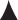# THE CALCULATOR SIDE OF THE FLIGHT COMPUTER

THE CALCULATOR SIDE OF THE FLIGHT COMPUTER

• The calculator side consists of a stationary portion with a flat circular portion attached. The circular portion can be turned.
1. The numbers along the outside of the stationary portion are referred to as the OUTER SCALE in this document.
1. The outer scale is used to represent distance, fuel, groundspeed, true airspeed, or corrected (true) altitude, depending on the calculation being performed.
2. The numbers on the edge of the rotating portion are referred to as the INNER SCALE in this document.
1. The inner scale is used to represent time, calibrated or indicated airspeed, and calibrated or indicated altitude, depending on the calculation being performed.
2. The number “60” on the inner scale has been replaced with a triangular-shaped arrow, referred to as the Index ().
1. This arrow is used as a reference to a rate, such as knots (nautical miles per hour) or gallons per hour.
3. In the center of the rotating portion are three “holes” (windows) used to compute corrected (true) altitude, density altitude, and true airspeed.
• Scale Values
1. The numbers on the outer and inner scales represent multiples of 10 of the values shown.
1. EXAMPLE: The number “20” on either scale may represent 0.2, 2.0, 20, 200, or 2,000.
2. On both the outer and inner scales you will notice that the number of tick marks, or graduations, vary between numbers.
1. EXAMPLES:
1. The first tick mark to the right of “10” may represent 10.1, 101, 1,100, etc.
2. The first tick mark to the right of “17” may represent 1.72, 17.2, 172, etc.
3. The first tick mark to the right of “35” may represent 3.55, 35.5, etc. The second tick mark to the right of “35” may represent 3.6, 36, 360, etc.
3. On the inner scale, minutes may be translated to hours and minutes by reference to the hour scale, which is below and inside the inner scale.
1. EXAMPLE: 120 min., or “12” on the inner scale is also 2 hr. as indicated by “2:00” below the “12.”
4. On the hour scale, the tick marks represent either 5 min. or 10 min.
1. EXAMPLE: Between “1:50” and “2:00” the tick mark represents 5 min. Between “4:00” and “4:30” each tick mark represents 10 min.
2. The tick marks on the inner scale can be used to supplement the hour scale.
• The calculator side of the flight computer is constructed so that any relationship, or ratio, between a number on the outer scale and a number on the inner scale will remain constant for all other numbers on both scales.
1. Rotate the inner circle so the “10” is opposite the “10” on the outer scale, and note that all the numbers around both circles are identical.
2. Next, rotate “20” under “10” on the outer scale; now all numbers on the inner scale are double those on the outer scale.